Histogram in R using ggplot2

• Difficulty Level : Easy
• Last Updated : 25 Feb, 2021

ggplot2 is an R Package that is dedicated to Data visualization. ggplot2 Package  Improve the quality and the beauty (aesthetics ) of the graph. By Using ggplot2 we can make almost every kind of graph In RStudio

A histogram is an approximate representation of the distribution of numerical data. In a histogram, each bar groups numbers into ranges. Taller bars show that more data falls in that range. A histogram displays the shape and spread of continuous sample data.

Histograms roughly give us an idea about the probability distribution of a given variable by depicting the frequencies of observations occurring in certain ranges of values. Basically, Histograms are used to show distributions of a given variable while bar charts are used to compare variables. Histograms plot quantitative data with ranges of the data grouped into the intervals while bar charts plot categorical data.

geom_histogram() function is an in-built function of ggplot2 module.

Approach

• Import module
• Create dataframe
• Create histogram using function
• Display plot

Example 1:

R

 set.seed(123)  # In the above line,123 is set as the # random number value# The main point of using the seed is to# be able to reproduce a particular sequence # of 'random' numbers. and sed(n) reproduces# random numbers results by seeddf <- data.frame(   gender=factor(rep(c(     "Average Female income ", "Average Male incmome"), each=20000)),   Average_income=round(c(rnorm(20000, mean=15500, sd=500),                           rnorm(20000, mean=17500, sd=600)))   )  head(df)  # if already installed ggplot2 then use library(ggplot2)library(ggplot2)  # Basic histogramggplot(df, aes(x=Average_income)) + geom_histogram()  # Change the width of binsggplot(df, aes(x=Average_income)) +         geom_histogram(binwidth=1)  # Change colorsp<-ggplot(df, aes(x=Average_income)) +        geom_histogram(color="white", fill="red")p

Output :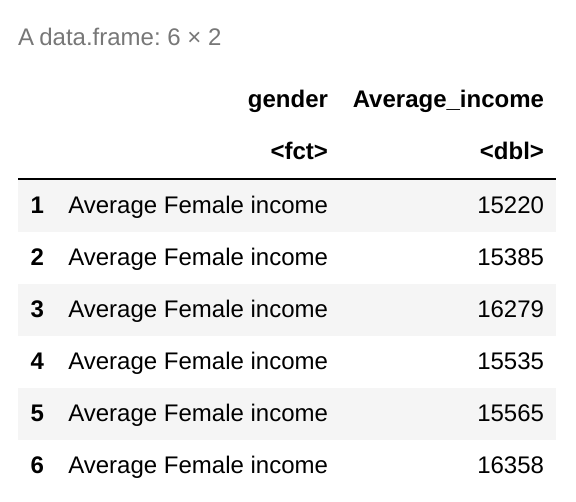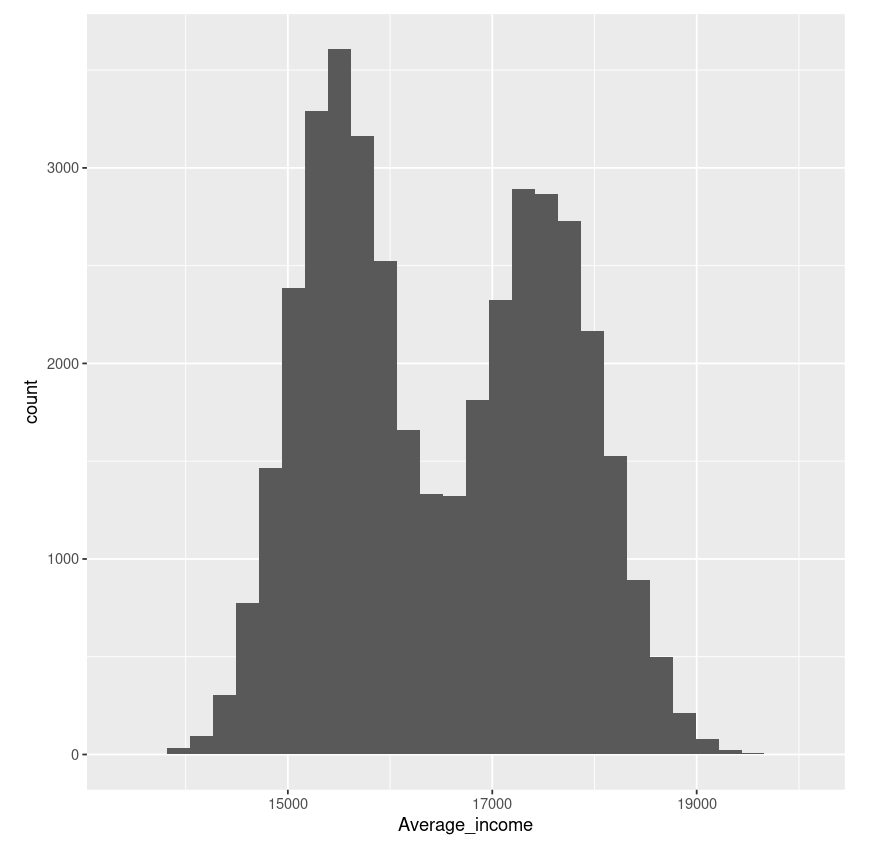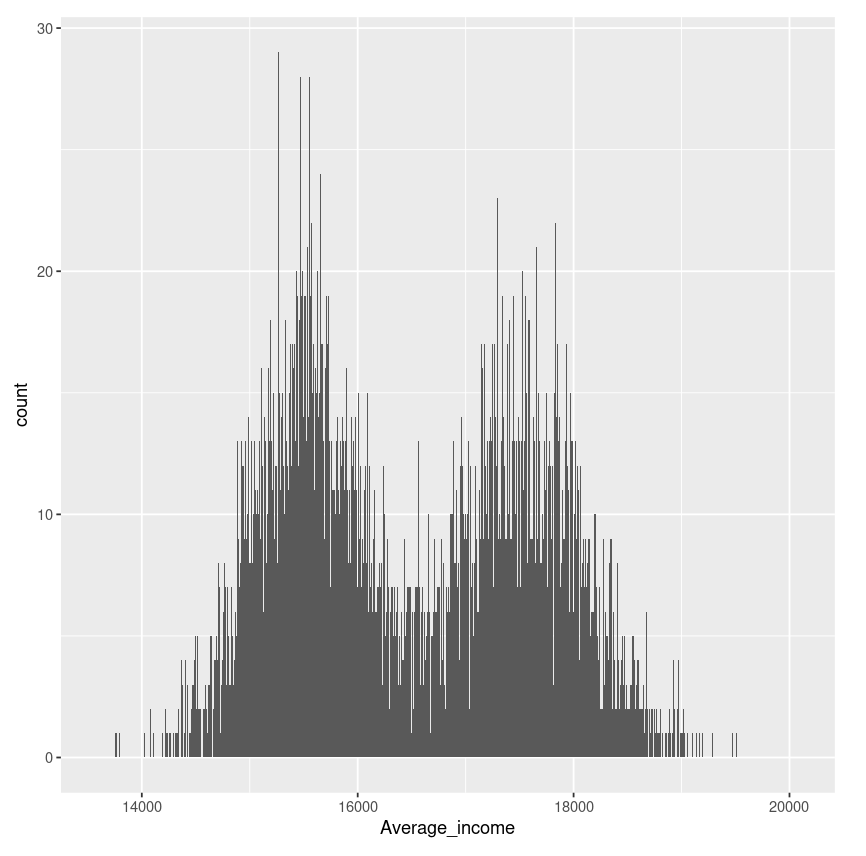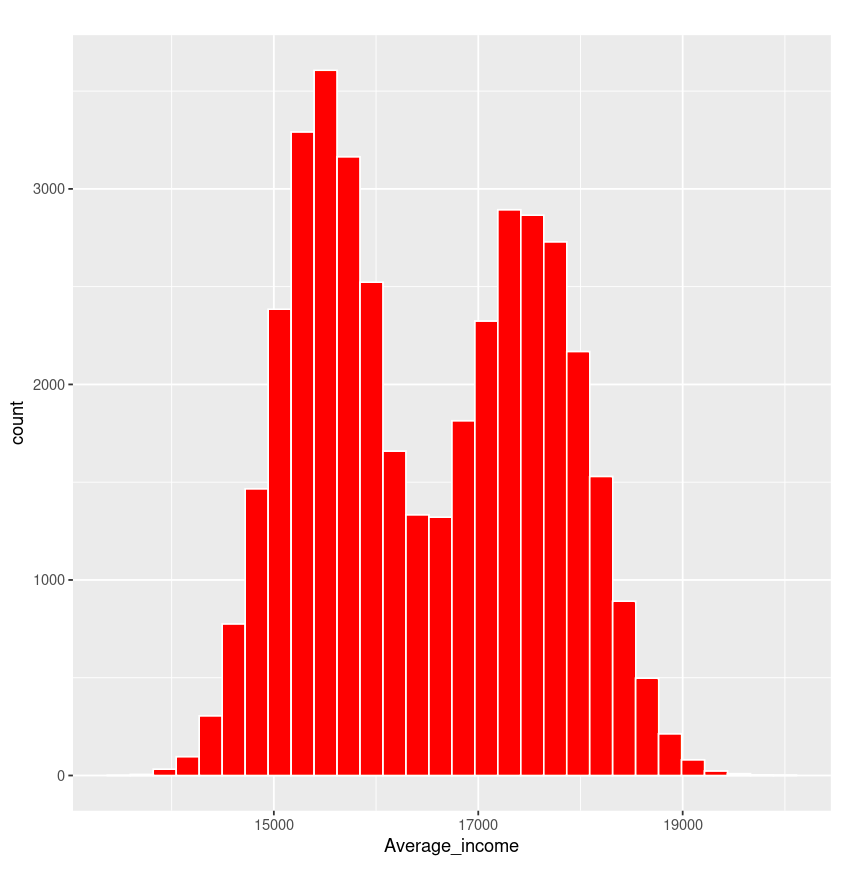Example 2:

R

 plot_hist <- ggplot(airquality, aes(x = Ozone)) +     # binwidth help to change the thickness (Width) of the bar    geom_histogram(aes(fill = ..count..), binwidth = 10)+     # name = "Mean ozone(03) in ppm parts per million "   # name is used to give name to axis     scale_x_continuous(name = "Mean ozone(03) in ppm parts per million ",                      breaks = seq(0, 200, 25),                      limits=c(0, 200)) +   scale_y_continuous(name = "Count") +     # ggtitle is used to give name to a chart   ggtitle("Frequency of mean ozone(03)") +   scale_fill_gradient("Count", low = "green", high = "red")  plot_hist

Output :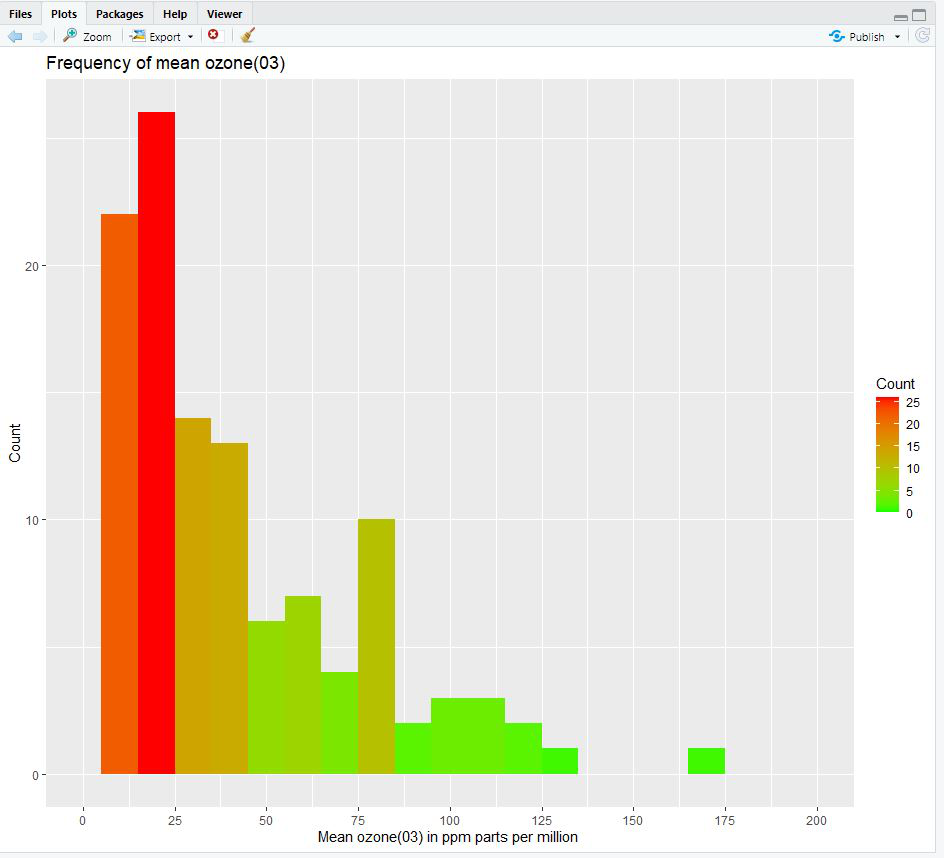My Personal Notes arrow_drop_up